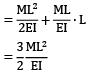Courses

# Test: Slope & Deflection Level - 1

## 15 Questions MCQ Test Strength of Materials (SOM) | Test: Slope & Deflection Level - 1

Description
This mock test of Test: Slope & Deflection Level - 1 for Mechanical Engineering helps you for every Mechanical Engineering entrance exam. This contains 15 Multiple Choice Questions for Mechanical Engineering Test: Slope & Deflection Level - 1 (mcq) to study with solutions a complete question bank. The solved questions answers in this Test: Slope & Deflection Level - 1 quiz give you a good mix of easy questions and tough questions. Mechanical Engineering students definitely take this Test: Slope & Deflection Level - 1 exercise for a better result in the exam. You can find other Test: Slope & Deflection Level - 1 extra questions, long questions & short questions for Mechanical Engineering on EduRev as well by searching above.
QUESTION: 1

### Other parameters being unchanged, if the span of a cantilever carrying end point load is doubled, the maximum slope is increased by

Solution:

(i)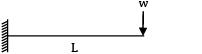θmax |1 =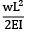(ii)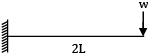θmax |2 =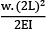θmax |2 = 4 × θmax|1

QUESTION: 2

### A cantilever beam of span L is carrying a uniformly distributed load of intensity w/unit length on the entire span. The deflection at the free end is given by

Solution: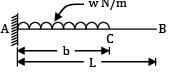θB =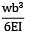QUESTION: 3

### A cantilever of length ‘L’ is carrying a uniformly distributed load ‘w’ per unit run for a distance of ‘b’ from fixed end. The slope at the free end is given as

Solution: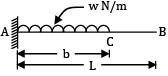θB =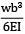QUESTION: 4

A cantilever beam of span L, uniform flexural rigidity EI is subjected to a unit couple at its free end. The deflection at the Centre of the beam is

Solution: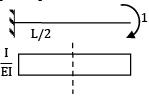δ =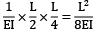QUESTION: 5

A cantilever of length of span ‘L’ carries a uniformly distributed load of w per unit length over its entire span. If its span is halved, then its slope will become

Solution: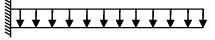θ1 =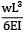θ2 =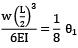QUESTION: 6

A cantilever beam of span ‘L’ is carrying a triangular load of zero intensity at its free end to W per unit length at its fixed end. the deflection at its free end will be

Solution: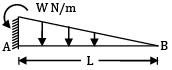YB =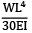QUESTION: 7

Slope at the end of the simply supported beam of span ‘L’ with uniformly distributed load w/unit length over the entire span is given by

Solution: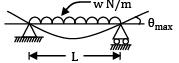θmax =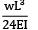QUESTION: 8

A beam of length ‘L’ simply supported at the ends carries a point load W at distance 'a' from the left end. Also L − a = b. The deflection under load is given by

Solution:
QUESTION: 9

A simply supported beam of span L carries a UDL of w. N m−1. What is the magnitude of concentrated load to be supplied at the centre of this beam which would produce the same deflection as the UDL?

Solution: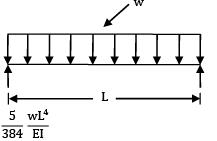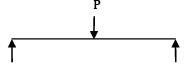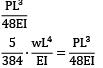P = 5/8 ∙ w ∙ L

QUESTION: 10

A simply supported beam of span L carries a concentrated load w at mid-span. The slope at the end is given by (EI = constant for total length)

Solution: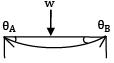θA = θB =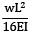QUESTION: 11

A simply supported beam of span ‘L’ carries a uniformly distributed load, 'w' per unit length over the entire span. The deflection at the center is 'y'. If the distributed load per unit length is doubled and also the depth of beam is doubled, the deflection at the center would be

Solution: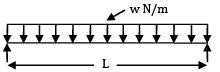δmax = y

w2 = 2w

d2 = 2d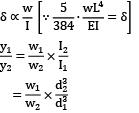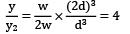y2 = y / 4

QUESTION: 12

If the actual beam has both ends fixed, then the ends of the conjugate beam will be

Solution:
QUESTION: 13

A cantilever beam AB is fixed at left end A and free at B. The corresponding conjugate beam will supports

Solution:
QUESTION: 14

A beam simply supported at the ends carries a load W at the Centre, causing deflection y. If the depth of the section of the beam is doubled, the deflection at the Centre will be

Solution: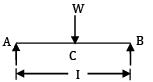Deflection at C:

y =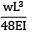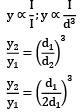y2 = 1/8 × y

QUESTION: 15

For cantilever beam shown in figure, the deflection at C due to a couple M applied at B is equal to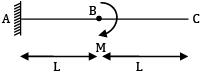Solution: ∆C= ∆B + QB ∙ L